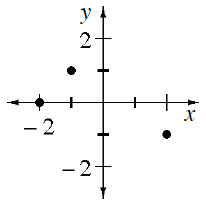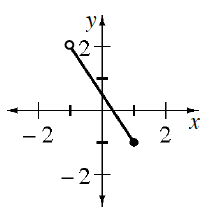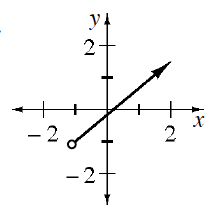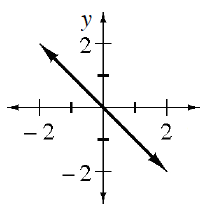### Home > INT3 > Chapter Ch1 > Lesson 1.2.2 > Problem1-84

1-84.

What are the domain and the range for each of the following functions? Write your answers using inequality symbols if possible.

1.Which $x$ values (domain) and $y$ values (range) are a part in the points?

1.Recall that open circles mean that the value is not included in the domain and range.

1.D: $x > -1$
R: $y > -1$

1.What do arrows on both sides of a line indicate?
Are there values it does not reach?